## Monday, March 26, 2007

### Depreciation of the One Hoss Shay

1.0 Introduction
Some economists have mistakenly thought that depreciation is merely a matter of capital goods wearing out:
"One of the advantages of the von Neumann model is that it can handle capital goods without fuss and bother. A nondepreciating capital good simply enters both as input and as output in the corresponding process. If the capital good depreciates 3 per cent per unit of time, 1 unit of the good may appear as input, and 0.97 unit as output." --Robert Dorfman, Paul A. Samuelson, and Robert M. Solow (1958: 382-383)
The above is an incorrect characterization of the von Neumann model. Von Neumann got depreciation right, unlike Dorfman, Samuelson, and Solow in that quotation. (Luigi Pasinetti points out this error.)

2.0 A Three-Period Example
2.1 Production
As an example of the correct calculation of depreciation, consider a machine of constant efficiency that lasts three production cycles. Each cycle takes a year. Table 1 summarizes the Constant-Returns-to-Scale production processes assumed to be in use for this example. The left five columns show inputs applied over the course of the year. The three right-most columns show outputs available at the end of the year. Oil is representative of inputs used up in producing the output, which the machine is an example of fixed capital. Suppose this machine, at whatever age, is only used in producing wheat; it is not used as an input in any production processes other than those shown in Table 1.
 1 New Machine & a Barrels Oil & l Person Yrs Produce 1 Quarter Wheat & 1 One-Year Old Machine 1 One-Year Old Machine & a Barrels Oil & l Person Yrs Produce 1 Quarter Wheat & 1 Two-Year Old Machine 1 Two-Year Old Machine & a Barrels Oil & l Person Yrs Produce 1 Quarter Wheat
This data illustrate the phenomenon of joint production. A process exhibits joint production when its output consists of more than one good. Because the von Neumann model is a model of general joint production, it "can handle capital goods without fuss or bother." Capital goods of different ages and history are distinguished as different goods; capital goods differing only in age are not treated as different quantities of identical goods. This is the point Dorfman, Samuelson, and Solow get wrong in the above quote.

A firm can be thought of as using all three of these production processes side by side. The managers of a firm will want to know how much to enter on thier books for old machines of different ages. They will also want to know the rate of (accounting) profits that they are making. The firm's book prices will be such that the firm is making the same rate of profits in all three process:(1)(2)(3)
where:
• p0 is the price of a new machine,
• p1 is the price of a one-year old machine,
• p2 is the price of a two-year old machine,
• pa is the price of a barrel oil,
• pw is the price of a quarter wheat,
• w is the wage, and
• r is the rate of profits.
A little algebra is of interest here. Multiply each of Equations 1, 2, and 3 by the appropriate power of (1 + r) to obtain Equations 4, 5, and 6:(4)(5)(6)
In summing, the prices of old machines drop out: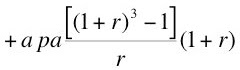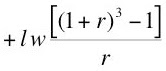(7)
Or: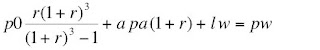(8)
In the price equations for an input-output system, Equation 8 can replace the system of equations specified in Displays 1, 2, and 3. Suppose the original system can be solved for prices, given the numeraire and, say, the rate of profits. Then the new system can be solved, as well. And the price of old machines does not appear in the system.

One can think about Equation 8 as follows. A wheat-producing firm buys a new machine that lasts for three production cycles. It doesn't make sense in calculating profit to charge the cost of the machine totally at the time it is bought. The later two production cycles make use of the services of the machine. The cost of the inputs for all three cycles should include some part of the cost of the machine. Equation 8 shows how much the machine should contribute to costs of the inputs of each year's production process, where the costs are assessed at the end of the year.

2.2 An Annuity
Consider an annuity, where (equal) payments are made at the end of three consecutive years. If the interest rate is such that the present value of this stream of payments is equal to the price, p0, of the annuity at the start of the first year, Equation 9 must hold:(9)
where x is the payment made at the end of the year. Summing the terms on the right, one obtains: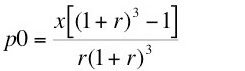(10)
That is, an annuity of:(11)
paid at the end of three years has a present value of p0.

The charge for the machine at the end of each production cycle, inclusive of profit and depreciation, is then the value of a fixed annuity paid at the end of each production cycle over the lifetime of the machine.

3.0 Generalizations and a Limit
The above analysis is easily generalized to a machine of constant efficiency that lasts for n years. The annual charge is: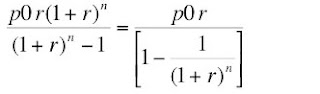(12)
One can also figure out the the price of the machine of constant efficiency at any age as a ratio of the price when new:(13)
Figure 1 shows this ratio, for various rates of profits, for the above example of a machine that lasts three years. (Sraffa provides a graph for machine that lasts 50 years, and the rate of profits in his example ranges up to 20%.) A machine that lasts forever would have a charge of p0 r. This is a charge only for profit; there is no depreciation in the case of a machine with an infinite lifespan. This conclusion correctly suggests that joint production provides a framework in which to analyze the rent of land.Figure 1: Dependence of Variation in Capital Value With Rate of Profits
The analysis can also be generalized to consider machines of varying technical efficiency throughout their age. An (industrial) plant might be analyzed as a collection of processes, where each process uses more than one kind of machine. These generalizations lead to more equations being appended to the system, as well as matching prices for machines of distinct ages and history. In principle, if the efficiency of the machines dependes on the history of the machines it is combined with as inputs in the processes, the number of equations can be countably infinite (Roncaglia 1978). The analysis must also be one of the choice of technique, where the technique includes the possibility of junking a machine while processes still remain that can use it. That is, the economic life of a machine may be shorter than its technical life.

It seems to me incredible that conventions for depreciation in practice could be the solutions of the general theory of joint production. One of the world’s foremost experts on the theory of joint production has this to say:
”Every economist knows that there is indeed much arbitrariness in accounting for depreciation or in the prices set for different derivatives from crude oil. Of course, competition always settles the matter somehow, but whereas it was easy – as de Quincey knew ... – to predict the value of shoes produced by a shoemaker in the nineteenth century, it is very difficult to predict on the basis of cost calculations how a modern refinery will recover its costs by setting administered prices for different refined products. Its rate of return for all its processes taken together can in general not deviate much from the rate of profit of the economy, but this observation does not explain how the relative prices of its individual products are set. Is this therefore another area where history must (as in the case of distribution) supplement theory because it is open-ended? The example of public goods as a form of joint social costs proves that there are cases of indeterminancy of possibly growing historical importance, but Sraffa has shown that there is a solution to the problem of the evaluation of joint products that does not require any explicit reference to the subjective elements of demand.” --Bertram Schefold (1989: 32)
The question becomes whether the number of cost-minimizing processes is equal to the number of products in the general case of joint production, except at flukes. I’m not convinced that the answer to this question is in the affirmative.

4.0 Conclusion
Suppose an economist claims that firms maximize profits. And that economist depicts depreciation as a technical datum, not dependent on prices and the rate of profits. Then, that economist has made a mistake in mathematics.

References
• Robert Dorfman, Paul A. Samuelson, and Robert M. Solow (1958). Linear Programming and Economic Analysis, Dover
• Luigi L. Pasinetti (1977). Lectures in the Theory of Production, Columbia University Press
• Alessandro Roncaglia (1978). Sraffa and the Theory of Prices (English translation), John Wiley & Sons
• Bertram Schefold (1989). Mr. Sraffa on Joint Production and Other Essays, Unwin-Hyman
• Piero Sraffa (1960). Production of Commodities by Means of Commodities: Prelude to a Critique of Economic Theory, Cambridge University Press

#### 10 comments:Anonymous said...

There are several definitions of depreciation: real, tax, profit statements. Real depreciation is the wear and tear of equipment and eventual obsolenscence. tax depreciation is what the govt allows and profit statement depreciation is what GAAP will allow for depreciation.

So in your example of the three machines the cost of the machines in each period would be p0/3 p1/3 and p2/3 So given all constraints being equal then a manager would use the first machine to it's fullest (if the cost rised of the machine) then the second and then the third. However I think there would be other variables that would change that decision.

anonymous Mike

Robert Vienneau said...

Mike, either you are just re-iterating a mistake exposed in my post or you miss the point of the post.

My post shows what depreciation would be if firms adopted accounting conventions consistent with profit-maximizing. The calculations necessary to properly figure depreciation require lots of data, and - which I already knew - conventional accounting precepts are inconsistent with my solution.

I suggest accounting conventions can be understood as heuristics or rules of thumbs. They should be analyzed in an evolutionary or institutional context - that is, in a particular non-optimizing approach. One of these years, I may get around to read John Maurice Clark's Studies in the Economics of Overhead Costs.Anonymous said...

I'm trying to understand it, but depreciation can have both simple and complex calculations.

What you did was the fancy calculation for NPV. And actually the method used for calculating it doesn't need the method used for calculating depreciation. It's only used in order to calculate the taxes that need to be paid and then it gets added back in.

Where it will make a difference is if a company uses cost markup to figure out a price and if that's your argument.

Firms underlying goal is maximizing cash flow, not profits per se.

Mike

YouNotSneaky! said...

This is a really nice example, though I gotta confess that I had some trouble with it until I read the poem. Maybe all those critics are right, maybe economics needs more poetry and less mathematics.

YouNotSneaky! said...

Having said that I got to agree with Anonimike. This is partially a matter of semantics, not a "mistake in mathematics". If by "depreciation" you mean the change in the economic value of a firm's capital stock, then yes, in general it will not be a technical datum independent of rate of profits (as far as prices go - your example can be greatly simplified without affecting anything by just dropping the oil and the labor. New machine produces wheat and an old machine, that's it. You can still make your point without the cumbersome algebra). However if by "depreciation" one simply means the change in the productive capacity of a machine then there's nothing that stops me from saying that depreciation in your example equals 0, 0, and 1 for each time period respectively. 0,0, and 1, are of course independent of rate of profits and prices.

A bit more interesting question is when the two definitions coincide or how we should treat depreciation in the real world.

First if rather then considering the One Hoss Shay of constant efficiency and finite life one takes a machine which lasts forever but each period produces a constant fraction of previous period's output (or has a constant probability of breaking down, risk attitudes aside) then you get some way to reconciling the two definitions. In this case the % change in the value of the firm's capital might still depend on r but will be independent of vintage. If r is then pinned down by some exogenous factor, like say the rate of time preference you get a constant depreciation rate, essentially a technical datum.

Second the brief but informative discussion towards the end suggests that the % change in the value of capital stock, while depending on profits and prices and vintage, will vary according to the actual nature of the technology and market structure, as well as plant size. In general there'll be no single formula which will apply to all profit maximizing firms. In that instance, for practical purposes (like taxes) it makes some sense to treat depreciation as a constant, particularly for long lived machines. Furthermore for modeling purposes in this sense the assumption of a "simple" depreciation rate independent of profits and vintage can be seen as a simplification in models where depreciation plays a secondary role.

I think your definition of depreciation is probably more "history of thought correct" but it also might be a good idea to seperate the concept of depreciation from that of a capital gain (though that's not exactly right either). At some level you do want to talk about the purely engineering properties of the production process, independent of market factors.Anonymous said...

good summary younotsneaky

It's interesting when I looked it up because economists and accountants give two different answers for what depreciation is. Economists give it as a change in value over time and accounts say that depreciation is the allocation of the costs over the period of time.

I think it's both but that each business has to decide which one is more pertinent to the issue.

However what I disagreed with you in your original post is where you said depreciation depends on price and profits. I would say no, that it depends on either looking it in terms of obsolencense (sp?) or allocating costs. If looking at allocating the cost, a business needs to decide if it's important to look at depreciation costs as an activity cost or an overhead cost.

anonymous MikeAdi the Austrian said...

Yes, these accounting methods of treating depreciation sound reasonable. Suppose that entrepreneur buys new machine for his plant which lasts n time periods. Entrepreneur has expectations concerning price of final product, price of new machines and cost of capital. So he also must somehow make a plan how to allocate capital costs amongst time periods.

And there are forms of investments which raise the demand for capital after original investments are made. Sunk costs dont matter after that and entrepreneur is willing to make new investments if they contribute to profits earned by using these machines.

It must be remembered that entrepreneur can freely use depreciation fund according to changing business environment so that automatic replacement of capital goods is not often true. So capital is not so immortal as said to be by Frank Knight.

Robert Vienneau said...

I don’t know what Mike is talking about. I wrote, in my post, that "It doesn’t make sense…to charge the cost of the machine totally at the time it is bought… The cost of the inputs for all three cycles should include some part of the cost of the machine." So I don’t know why Mike suggests I reject the accountants who say "that depreciation is the allocation of the costs over the period of time." And I should think accountants should be able to tell a manager how much a one-year old machine must sell for to cover its costs. So I don’t see the distinction between what accountants and economists say.

And I find Radek’s comments puzzling as well. As far as I can see, Table 1 in my post "talk[s] about the purely engineering properties of the production process." I am aware of the radioactive decay model of depreciation. If one wants to claim a model is a simplification, one should point to an analysis where the simplification results from ignoring some negligible effect or some second-order term in the analysis. (I think, for example, of treating sine as the identity function for small angles in the determination of the period of a pendulum.) Also Radek, I must not have been clear if you think that depreciation only applies to the total value of the firm’s capital. The general analysis I pointed to at the end of my post determines the price, given the vintage, of each of the many machines that might make up a plant. The numerical example suggests this insofar as two kinds of capital goods, machines and oil, are depreciated. Granted, I did not point out that oil’s loss of 100% of its value each year is a trivial case of depreciation.

For Adi, I'll note Sraffa explicitly analyzes machines left over from past years, but no longer produced.Anonymous said...

I just meant economists in general in the comment about differences in definitions.

Depreciation along with other items, profit is a very arbritary number.

YouNotSneaky! said...

My beef wasn't with the post itself, just with your unjustified conclusion:

"Suppose an economist claims that firms maximize profits. And that economist depicts depreciation as a technical datum, not dependent on prices and the rate of profits. Then, that economist has made a mistake in mathematics."

If the economist by depreciation means "the decline in the physical productive capacity of equipment" then this is obviously independent of prices and rate of profits - an engineering datum. In some cases, in simple models whose purpose is not to analyze the full gamut depreciation-related issues, this decline may be modeled simply with the single parameter rather a table as in your example.

But what you're talking about is the change in the market value of a firm's equipment - which isn't exactly depreciation but well, the change in the market value of a firm's equipment. Of course once you put that "market value" in there, one wouldn't expect it to be independent of rate of profit and vintage.

I understand your point about oil and machines and the general case. Like I said previously, this IS a very nice example of how these issues can be dealt with in context of joint production.